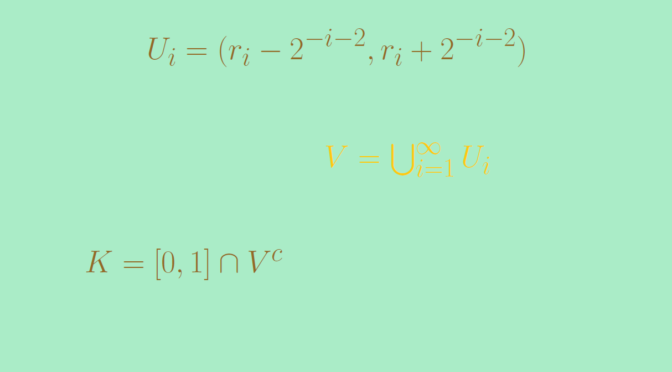# Totally disconnected compact set with positive measure

Let’s build a totally disconnected compact set $$K \subset [0,1]$$ such that $$\mu(K) >0$$ where $$\mu$$ denotes the Lebesgue measure.

In order to do so, let $$r_1, r_2, \dots$$ be an enumeration of the rationals. To each rational $$r_i$$ associate the open interval $$U_i = (r_i – 2^{-i-2}, r_i + 2^{-i-2})$$. Then take $\displaystyle V = \bigcup_{i=1}^\infty U_i \text{ and } K = [0,1] \cap V^c.$ Clearly $$K$$ is bounded and closed, therefore compact. As Lebesgue measure is subadditive we have $\mu(V) \le \sum_{i=1}^\infty \mu(U_i) \le \sum_{i=1}^\infty 2^{-i-1} = 1/2.$ This implies $\mu(K) = \mu([0,1]) – \mu([0,1] \cap V) \ge 1/2.$ In a further article, we’ll build a totally disconnected compact set $$K^\prime$$ of $$[0,1]$$ with a predefined measure $$m \in [0,1)$$.